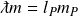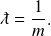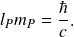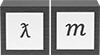# Length-mass symmetry

The product of length and mass is conserved in both particles and fields. Accordingly, the wavelength of an elementary particle has a corresponding inverse quantity of inertial mass.

Length-mass symmetry is shown in the illustration below as the correlation of length and mass potentials, where length potential is the inverse of its unit value. The equivalence of length and mass potential means that a dimensionless proportionality operator in one unit dimension can be applied to the other.

The wavelength operator quantifies a particle’s wavelength and inertial mass in the ratios

## Derivation

Elementary forms of the Compton and de Broglie wavelength formulas give the following equality for all elementary particles, whether or not they have rest massUsing a natural unit value of 1 for the Planck length and Planck mass gives the inverse relationship between wavelength and inertial massSince 𝑙𝑃𝑚𝑃 is constant, the formula can be stated in SI units as== 3.52 x 10-43 kgm

The conserved quantity 3.517 672 94… x 10-43 kgm has an exact value under the May 2019 redefinition of the International System of Units, in which Planck’s constant and the speed of light were given exact values. The exact value of 𝑙𝑃𝑚𝑃 is given by the formulaThe inverse relationship between wavelength and inertial mass means that the product of Planck length and Planck mass is not only the maximum length-mass potential, but is a conserved quantity for every combination of wavelength and inertial mass. The following table summarizes the length-mass symmetry

ƛ𝑚 = 𝑙𝑃𝑚𝑃

### Potential### Invariant### Conserved quantity

3.52 x 10-43 kgm

The length-mass symmetry is reflected in non-relativistic quantities of particle wavelength and inertial mass, shown in the following table using the mechanical properties of photons. The table applies the wavelength operator 𝑙𝑃 / ƛ to the Planck length and mass, producing observable quantities of wavelength and inertial mass proportional to the Planck scale. Quantities calculated using New Foundation Model operators are equivalent to historical formulas in which Planck’s constant is used.

** The table shows the length form of the wavelength operator but mass and time forms can also be used

** Natural formulas shown in the table are the same for particles with and without rest mass

The length-mass symmetry is used in a number of equations to find wavelength from mass, and mass from wavelength—including formulas for the Compton wavelength, de Broglie wavelength, and Schwarzschild radius.

The quantity 3.52 x 10-43 kgm is an important physical constant in the conservation of length-mass. The consistency of this relationship across the different elementary particles of the standard model suggests that a standard particle unit may underlie each of the elementary particles of the standard model. Because the length-mass relationship is conserved for individual particles–reflecting the quantization of particle fields—the New Foundation Model refers to this constant as the quantum constant.

A more natural representation of physics quantifies momentum in unit dimensions of M and energy in unit dimensions LMT-1. In this representation, the quantum constant is equal to Planck’s constant.

Length-mass symmetry